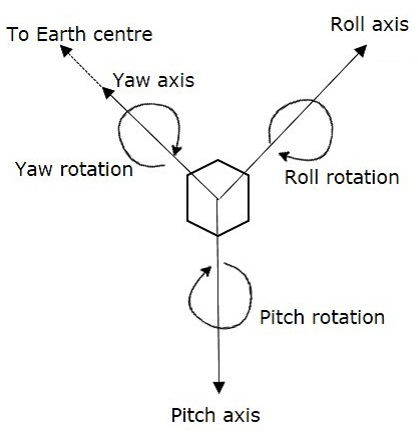# Satellite Communication - AOC Subsystem

We know that satellite may deviates from its orbit due to the gravitational forces from sun, moon and other planets. These forces change cyclically over a 24-hour period, since the satellite moves around the earth.

Altitude and Orbit Control (AOC) subsystem consists of rocket motors, which are capable of placing the satellite into the right orbit, whenever it is deviated from the respective orbit. AOC subsystem is helpful in order to make the antennas, which are of narrow beam type points towards earth.

We can make this AOC subsystem into the following two parts.

• Altitude Control Subsystem
• Orbit Control Subsystem

Now, let us discuss about these two subsystems one by one.

## Altitude Control Subsystem

Altitude control subsystem takes care of the orientation of satellite in its respective orbit. Following are the two methods to make the satellite that is present in an orbit as stable.

• Spinning the satellite
• Three axes method

### Spinning the satellite

In this method, the body of the satellite rotates around its spin axis. In general, it can be rotated at 30 to 100 rpm in order to produce a force, which is of gyroscopic type. Due to this, the spin axis gets stabilized and the satellite will point in the same direction. Satellites are of this type are called as spinners.

Spinner contains a drum, which is of cylindrical shape. This drum is covered with solar cells. Power systems and rockets are present in this drum.

Communication subsystem is placed on top of the drum. An electric motor drives this communication system. The direction of this motor will be opposite to the rotation of satellite body, so that the antennas point towards earth. The satellites, which perform this kind of operation are called as de-spin.

During launching phase, the satellite spins when the small radial gas jets are operated. After this, the de-spin system operates in order to make the TTCM subsystem antennas point towards earth station.

### Three Axis Method

In this method, we can stabilize the satellite by using one or more momentum wheels. This method is called as three-axis method. The advantage of this method is that the orientation of the satellite in three axes will be controlled and no need of rotating satellite’s main body.

In this method, the following three axes are considered.

• Roll axis is considered in the direction in which the satellite moves in orbital plane.

• Yaw axis is considered in the direction towards earth.

• Pitch axis is considered in the direction, which is perpendicular to orbital plane.

These three axes are shown in below figure.Let XR, YR and ZR are the roll axis, yaw axis and pitch axis respectively. These three axis are defined by considering the satellite’s position as reference. These three axes define the altitude of satellite.

Let X, Y and Z are another set of Cartesian axes. This set of three axis provides the information about orientation of the satellite with respect to reference axes. If there is a change in altitude of the satellite, then the angles between the respective axes will be changed.

In this method, each axis contains two gas jets. They will provide the rotation in both directions of the three axes.

• The first gas jet will be operated for some period of time, when there is a requirement of satellite’s motion in a particular axis direction.

• The second gas jet will be operated for same period of time, when the satellite reaches to the desired position. So, the second gas jet will stop the motion of satellite in that axis direction.

## Orbit Control Subsystem

Orbit control subsystem is useful in order to bring the satellite into its correct orbit, whenever the satellite gets deviated from its orbit.

The TTCM subsystem present at earth station monitors the position of satellite. If there is any change in satellite orbit, then it sends a signal regarding the correction to Orbit control subsystem. Then, it will resolve that issue by bringing the satellite into the correct orbit.

In this way, the AOC subsystem takes care of the satellite position in the right orbit and at right altitude during entire life span of the satellite in space.